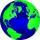Fish tank

A fish tank at a pet store has 8 zebra fish. In how many different ways can George choose 2 zebra fish to buy?

Result

n =  28

Solution:Leave us a comment of this math problem and its solution (i.e. if it is still somewhat unclear...):Be the first to comment!To solve this verbal math problem are needed these knowledge from mathematics:

Would you like to compute count of combinations?

Next similar examples:On the menu are 12 kinds of meal. How many ways can we choose four different meals into the daily menu?
2. ConfectioneryThe village markets have 5 kinds of sweets, one weighs 31 grams. How many different ways a customer can buy 1.519 kg sweets.
3. ChordsHow many 4-tones chords (chord = at the same time sounding different tones) is possible to play within 7 tones?
4. TrinityHow many different triads can be selected from the group 43 students?
5. DivisionDivision has 18 members: 10 girls and 6 boys, 2 leaders. How many different patrols can be created, if one patrol is 2 boys, 3 girls and 1 leader?
6. CommitteesHow many different committees of 6 people can be formed from a class of 30 students?
7. ExaminationThe class is 21 students. How many ways can choose two to examination?
8. A studentA student is to answer 8 out of 10 questions on the exam. a) find the number n of ways the student can choose 8 out of 10 questions b) find n if the student must answer the first three questions c) How many if he must answer at least 4 of the first 5 que
9. TeamsHow many ways can divide 16 players into two teams of 8 member?
10. CombinationsHow many elements can form six times more combinations fourth class than combination of the second class?
11. CombinationsFrom how many elements we can create 990 combinations 2nd class without repeating?
12. BlocksThere are 9 interactive basic building blocks of an organization. How many two-blocks combinations are there?
13. 2nd class combinationsFrom how many elements you can create 4560 combinations of the second class?
14. Calculation of CNCalculate: ?
15. Theorem proveWe want to prove the sentence: If the natural number n is divisible by six, then n is divisible by three. From what assumption we started?
16. CardsThe player gets 8 cards of 32. What is the probability that it gets a) all 4 aces b) at least 1 ace
17. One greenIn the container are 45 white and 15 balls. We randomly select 5 balls. What is the probability that it will be a maximum one green?